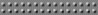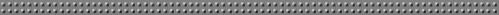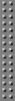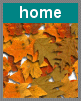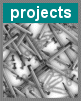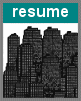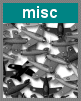Computer Graphics Project Project Source hw.08.tar.gz Project Part 1 - Samples Part 1 demonstrates the use of random unit vectors. The small spheres that "grow" on the outside of the larger sphere are randomly placed by using a random unit vector. It's crazy! The random unit vectors are produced as follows; SbVec3f vUnit; int lengthOK = 0; while (!lengthOK) {    // vx in [-1,1], vy in [-1,1], vz in [-1,1]    vUnit = randVec3f(-1.0, 1.0);    lengthOK = (vLen > 0.0 && vLen <= 1.0); } vUnit.normalize();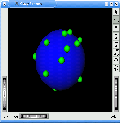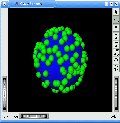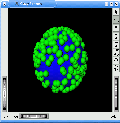Project Part 2, 3, and 4 - Samples Part 2 builds a scene with a dragger. Random unit vectors are used to produce arrows from the dragger randomly. If the path of an arrow intersects a triangle in the scene, then an arrow is drawn from the intersection point back to the dragger. As well, another random unit vector is used to draw an arrow in a random direction from that point. Part 3 adds to Part 2 by implementing a recursive ray tracer. Now when an intersection point is found, a random unit vector is used to pick a random path from the intersection point. This new direction is again followed for further intersections. Part 4 adds to Part 2 and Part 3 by determining the arrow color based off the light coming into the intersection point. Lambert Shading is used to help determine this triangle color using the light emmitted from other rays. The follow is how Lambert Shading is implemented; vinp = -vUnit; // vUnit is the random unit vector from p to q. Loutp = diffuseColor * Linp * vinp.dot(normalp); // red Loutp = diffuseColor * Linp * vinp.dot(normalp); // green Loutp = diffuseColor * Linp * vinp.dot(normalp); // blue The following images are samples from the additive implementation of Parts 2, 3, and 4!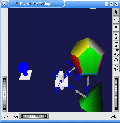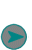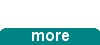projects